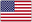# Core Notions of Logic

0    25 Datenblatt

FrageAntwortenProposition
A fact in the world; a state of affairs.
The same proposition can be expressed in multiple different sentences.
Sentence
A linguistic entity which expresses a proposition.
In logic, we only care about declarative sentences.
Argument
A collection of sentences, some of which are premises, and exactly one of which is the conclusion.
Arguments should exhibit some connection between the premises and the conclusion.
Validity
A property of arguments; an argument is valid if, and only if, every time all the premises are true it is impossible for the conclusion to be false.
Only arguments can be valid, for validity expresses a relationship between premises and the conclusion in an argument.
Soundness
A property of argument; an argument is sound if, and only if, it is a valid argument with true premises.
Soundness requires both that the argument is valid and that all the premises of the argument must be true.
A valid argument can have a false conclusion.
True
Only if some or all of the premises are false. If the all premises were true and the conclusion still false, the argument can't be considered a valid truth-function.
A sound argument can have a false conclusion.
False
Due to the properties of soundness, the truth of the premises would have to be transmitted to the conclusion's own truth-value by virtue of the argument's form.
A valid argument can have false premises.
True
Validity is more concerned about the form of the argument rather than the empirical truth of the premises or conclusion.
Sequent
A finite, possibly empty set of formulae, separated from each other by commas, and then followed by a single formula which is separated from the rest by the turnstile (|–).
The set of formulae left of the turnstile are premises, and there may be any number of them. The formula right of the turnstile is the conclusion, and all sequents must have this. The comma is not a connective, and a colon may take the place of turnstile.
Entailment
Entailment is a relation in the metalanguage. It represents logical consequence through probability and derivability. It is represented by the turnstile.
The conclusion of a sequent really does follow logically from its premises in PL.
"In the formal language PL, this conclusion is a logical consequence of these premises."
Material Implication
Material implication is a relation in the object language. It represents the claim that the consequent of a given conditional is not false when its antecedent is true. It is represented by the arrow.
If the consequent is false when the antecedent is true, the conditional itself will not be true.
"Having assumed the antecedent, the consequent can be shown to hold."
Deduction Theorem for PL
How does this demonstrate the relation between entailment and material implication?
「If X, A |- B then X |- A --> B」and 「If X |- A --> B then X, A |- B」
「A |- B if and only if |- A --> B」
If a set of PL formulae together with a particular PL formula entails another formula, then the original set of formulae entails a conditional with the first particular formula as antecedent and the second as consequent.
Bracket
Provide the left-handed and right-handed versions.
'(', ')'
Logical connective
'&', 'v', '~', '-->', '< -- >'
Sentence-letter
P, Q, R...
P, P`, P``...
Symbol
Any mark already defined
Sequence of symbols
An ordered list of symbols
Formula
A sequence of symbols
1st Rule of Well-Formedness
Any sentence-letter is an atomic well-formed formula.
2nd Rule of Well-Formedness
~A is a well-formed formula if A is a well-formed formula.
3rd Rule of Well-Formedness
(A & B) is a well-formed formula if A and B are well-formed formulae.
4th Rule of Well-Formedness
(A v B) is a well-formed formula if A and B are well-formed formulae.
5th Rule of Well-Formedness
(A --> B) is a well-formed formula if A and B are well-formed formulae.
6th Rule of Well-Formedness
(A < -- > B) is a well-formed formula if A and B are well-formed formulae.
7th Rule of Well-Formedness
Justify how, for example, A & B is a well-formed formula despite lacking brackets.
Nothing else [not sanctioned by Rules 1–6] is a well-formed formula.
Brackets are used to disambiguate formula, but no ambiguity is introduced by omitting the outermost bracket pair. Therefore, such bracket proliferation can be ignored; A & B is thus identical to (A & B).